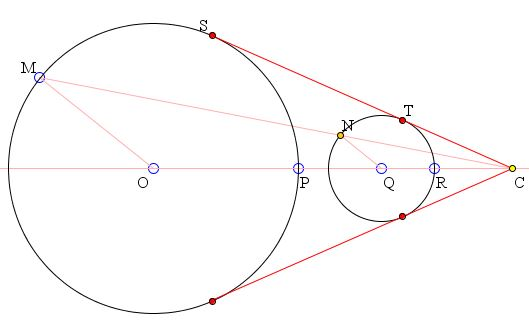# Common Tangents to Two Circles II What is this about? A Mathematical Droodle

### If you are reading this, your browser is not set to run Java applets. Try IE11 or Safari and declare the site https://www.cut-the-knot.org as trusted in the Java setup.What if applet does not run?

(Points O, P, Q, R move horizontally. The diagram can also be dragged as a whole.)

ExplanationTwo circles may intersect or lie entirely one within or without the other. If one of the circles lies inside the other, the two have no common tangents. Otherwise, the two circles do have common tangents. The number of the tangents may be 2, 3, or 4 depending as to whether the circles intersect in 2, 1 (i.e. they are tangent to each other), or 0 points.

When the number of tangents is 2, they are both external in the sense that, for each, the points of tangency with the circles lie on the same side of the circles' center line OQ.

When the circles touch each other, the line through the point of tangency perpendicular to their center line is also tangent to the circles.

When the circle are away from each other and do not have common points, there is an additional pair of common tangents that intersect the center line between the centers of the circles. These are known as the internal tangents of the two circles.

(Depending on the relative position of points P and R that determine the size of the circles, the tangents shown may be external or, if the configuration allows, internal to the circles.)

### If you are reading this, your browser is not set to run Java applets. Try IE11 or Safari and declare the site https://www.cut-the-knot.org as trusted in the Java setup.What if applet does not run?

The applet illustrates one possible way of constructing the common tangents to the two circles. Like another approach, the method is based on the construction of a tangent to a circle from a point outside the circle. This uses the fact that the radius-vector to the point of tangency is perpendicular to the tangent.

Now, any two circles are similar. They may have 1 or 2 centers of similitude. Centers of similitude lie on the line joining the centers of the circles. Any common tangent to two circles passes through one of the centers of the similitude. This suggests a construction: first find a center of the similitude of the two given circles and from there draw tangents to each of them. (The last step is common to another construction.)

In order to find a center of similitude, choose a point (M) on one of the circles, say O, and draw a line through Q parallel to OM. The points of intersection will serve as images of M under the similarity transformation. The line joining M to either of the points will pass through through a center of similitude.